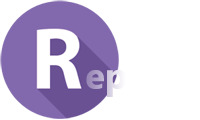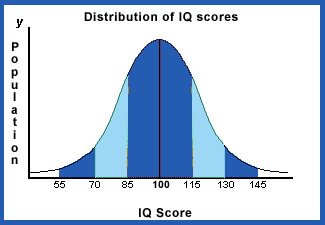# IQ Test Labs

Discover your intellectual strengths### Sample personalized IQ report for Jane

Thank you for taking the test.

Your age adjusted IQ score is 106.

The average score for all test takers is 100.

You scored higher than 64 % of all the people that took this test.

General statistics:

Total number of questions: 30

Questions answered correctly: 16

Questions answered incorrectly: 14

Percentage correct answers: 53 %The IQ Test Labs scale is equivalent to the Wechsler scale

(mean=100 and standard deviation=15)

The bell curve (also called a "normal curve" or "normal distribution") is a graph that shows approximately how much of the population falls into each IQ range. In theory, if we tested everyone in the world with a traditional IQ test, most people would score in the " Average" range. A smaller number would score moderately below average and moderately above average. Very high and very low scores are rare.

The scores and percentiles above apply to tests that have a standard deviation of 15 points. The Stanford Binet Fourth Edition (Binet FE) has a standard deviation of 16 and the Wechsler scales (such as the WPPSI-R, the WISC-III, and the WAIS-III) have a standard deviation of 15. Therefore, for different scales the percentages may vary.

Click here to convert your score to the Cattell and Stanford-Binet scale.